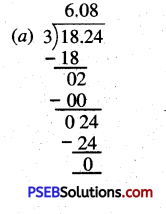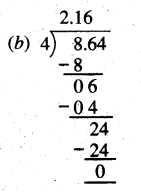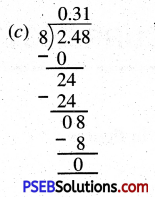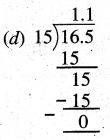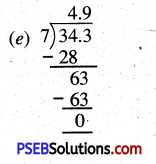# PSEB 5th Class Maths Solutions Chapter 4 Fractions Ex 4.9

Punjab State Board PSEB 5th Class Maths Book Solutions Chapter 4 Fractions Ex 4.9 Textbook Exercise Questions and Answers.

## PSEB Solutions for Class 5 Maths Chapter 4 Fractions Ex 4.9

Question 1.
Find the product of the following decimal numbers :
(a) 5.15 × 6
(b) 52.4 × 2
(c) 0.31 × 5
(d) 9.05 × 0.2
(e) 7.24 × 2.3.
Solution:
(a) 5.15 × 6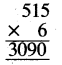5.15 × 6 = 30.90

(b) 52.4 × 2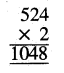52.4 × 2 = 104.8

(c) 0.31 × 5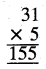0.31 × 5 = 1.55

(d) 9.05 × 0.2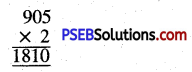9.05 × 0.2 = 1.810

(e) 7.24 ×2.3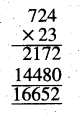7.24 × 2.3 = 16.652Question 2.
Find the division of the following decimal numbers :
(a) 18.24 ÷ 3
(b) 8.64 ÷ 4
(c) 2.48 ÷ 8
(d) 16.5 ÷ 15
(e) 34.3 ÷ 7.
Solution: